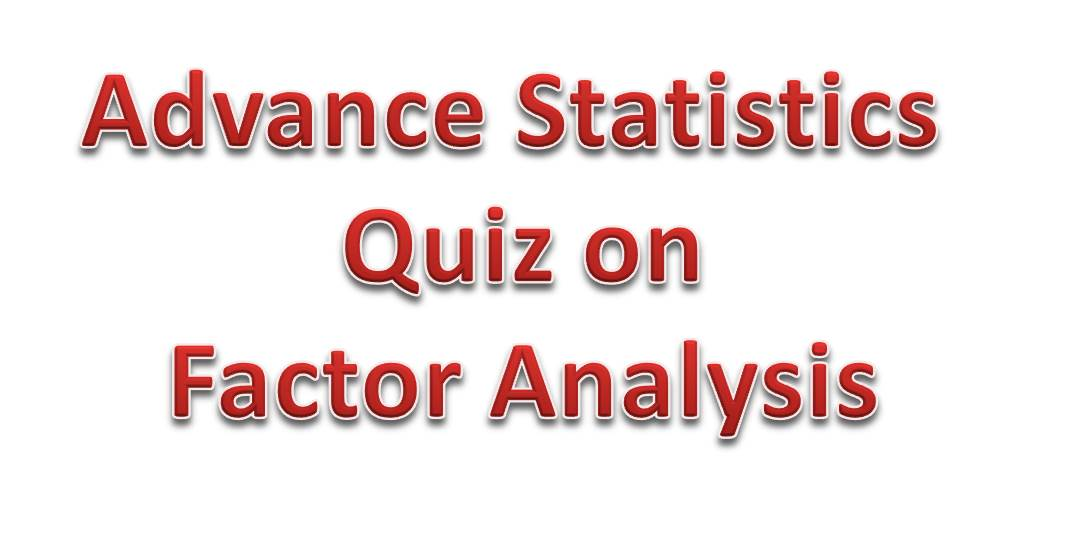# Advanced Statistics Quiz 9 – Concepts from Factor Analysis and PCAToday’s quiz would be based on the concept of Factor Analysis. I guess you will find it clear and interesting.

Question 1: Briefly explain Factor Analysis?
Factor analysis is a statistical procedure used to draw inferences on quantities that cannot be quantified numerically. Quantities such as intelligence, friendliness and patriotism.

Question 2: What is the goal of Factor Analysis?
The objective a factor analysis is to describe the correlation between p measured attributes in terms of variation in few underlying unobservable factors.

Question 3: Explain Communality and Uniqueness in Factor Analysis
These explains the variation caused by the different factors under consideration. The portion of the variance that is contributed by the k common factors is the communality and the portion that is not explained by the common factor is the uniqueness also known as specific variance

Question 4: Outline the various methods of carrying out Factor Analysis problem

• Maximum Likelihood Method
• Least Squares Method
• Principal Components
• Alpha Factoring
• Image Factoring

Question 5:  What is the concept of Rotation in Factor Analysis?
Rotation is the process of obtaining a different set of loadings by multiplying  the loadings by an orthogonal matrix chosen based on a specific criteria.

Question 6: Outline and explain the types of rotations you know?
Varimax: Orthogonal rotation that minimizes the number of variables that have high loadings on each other. It simplifies interpretation of factors
Quartimax: A rotation mentod that minimizes the number of factors needed to explain each variable. It simplifies interpretation of observed variable
Equamax:A mixed rotation method which is a combination of the Varimax and the Quatimax methods.
Direct Oblimin: Non-orthogonal rotation
Promax Rotation: Non-orthogonal rotation  that allows correlation of factors.

Question 7: What is Keiser-Meyer-Olkin(KMO) Test used for?
The KMO is a measure of how suitable the available data is to be used for Factor Analysis. The value of the KMO statistic ranges from 0 to 1.

Question 8: What is Principal Component Analysis(PCA)?
PCA is a variance-maximization procedure that aims are transforming a set of features into  few principal components

Question 9: How if Principal Component Analysis Performed?

• Compute teh sample covariance matrix
• Compute the eigenvalues
• Choose a dimension k
• Define the dimension reduced data

Question 10: Briefly Explain Bartlett’s Test of Sphericity
Bartlett’s test is a measure of sampling adequacy and relates to the significance of the study and just like the KMO, test how suitable the data is.

Take some time to get used to these concepts in your own word and I would like to thank you or reading!